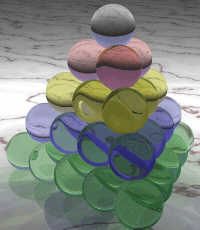# Tetrahedral Number SequenceThis is the Tetrahedral Number Sequence:

1, 4, 10, 20, 35, ...

We can understand it better when we think of a stack of marbles in the shape of a Tetrahedron.

Just count how many marbles are needed for a stack of a certain height.

• For height=1 we only need 1 marble
• For height=2, we need 4 marbles (1 at the top and 3 below)
• For height=3 we need 10 marbles.
• For height=4 we need 20 marbles.
• For height=5 we need 35 marbles.
• How many for height=6 ... ?

## Triangular and Tetrahedral Numbers

Each layer in the tetrahedron of marbles is actually part of the Triangular Number Sequence (1, 3, 6, etc). And both the triangular numbers and the tetrahedral numbers are on Pascal's Triangle.

This table shows the values for the first few layers:

n Triangular Number Tetrahedral Number
(Height) (Marbles in Layer) (Total Marbles)
1 1 1
2 3 4
3 6 10
4 10 20
5 15 35
6 21 56

Looking at the numbers we see something interesting: when we take any number and add the number before it and to the left we get the next number in the sequence. (For example 4+6=10).